"A pinch of probability is worth a pound of perhaps."
- James Thurber (American humorist and cartoonist)

# About the Probability and Statistics App

## Covered Topics

In the area of probability, the app covers combinations and permutations, the difference between probability and odds, Pascal's Triangle, and random numbers (you can even use it to generate your own random numbers). Real life probabilities are illustrated using games like cards dice and Keno. The Monty Hall problem is also covered. Eight famous probability distributions will be covered upon completion of the app, but currently only the Normal and Lognormal distributions sections of the app have been built.

In the area of statistics, the app will cover 1- and 2-dimensional data, the three Pythagorean Means, measures of central tendency, measures of dispersion, skewness, kurtosis, stem-and-leaf plots and box-and-whisker plots. In addition, the completed app will cover applications of statistics to Decision Theory, Curve Fitting, and Time Series data.

The app also contains an extensive glossary of terms relating to Probability and Statistics.

### Probability

Below are some thumbnails that you can click to get a better idea of what you can do with Probability.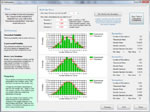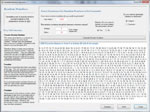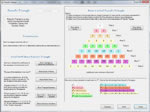Permutations and
Combinations
Roll Dice Play Keno Generate
Random Numbers
Pascal's
Triangle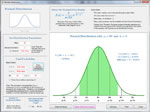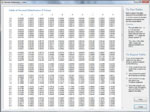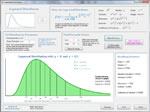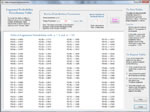Binomial
Theorem
Normal
Distribution
Table of Normal
Distribution z-Values
Lognormal
Distribution

Table of Lognormal
Percentile Values

### Statistics

The Statistics portion of the app is early in its development. More information will be provided as the app develops.